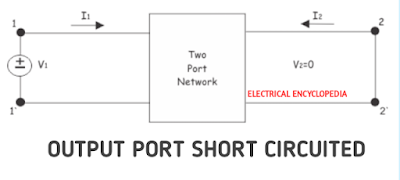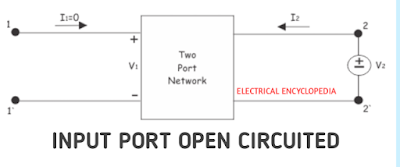# H Parameters (Hybrid Parameters) in Two Port Networks

0
638

### CASE-1 SHORT CIRCUITING THE OUTPUT PORT.

#### If the Output port of the two port network is  short circuited then output voltage is zero, i.e. V2 = 0.### CASE-2 OPEN CIRCUITING THE INPUT PORT.

#### If the Input port of the two port network is open circuted then the input current is zero, i.e. I1 = 0.#### EQUIVALENT CIRCUIT OF Y-PARAMETERREAD HERE  Registers & Shift Registers: Definition, Function & Examples